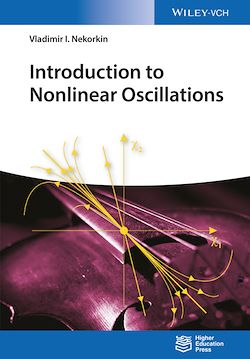# Introduction to Nonlinear Oscillations

-English
264 Pages

Description

A systematic outline of the basic theory of oscillations, combining several tools in a single textbook. The author explains fundamental ideas and methods, while equally aiming to teach students the techniques of solving specific (practical) or more complex problems.

Following an introduction to fundamental notions and concepts of modern nonlinear dynamics, the text goes on to set out the basics of stability theory, as well as bifurcation theory in one and two-dimensional cases. Foundations of asymptotic methods and the theory of relaxation oscillations are presented, with much attention paid to a method of mappings and its applications.

With each chapter including exercises and solutions, including computer problems, this book can be used in courses on oscillation theory for physics and engineering students. It also serves as a good reference for students and scientists in computational neuroscience.

Subjects

##### Mathematical physics

Informations